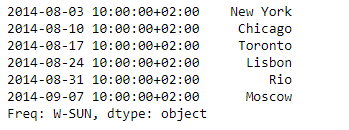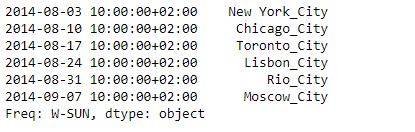Open in App
Not now

# Python | Pandas Series.transform()

• Last Updated : 05 Feb, 2019

Pandas series is a One-dimensional ndarray with axis labels. The labels need not be unique but must be a hashable type. The object supports both integer- and label-based indexing and provides a host of methods for performing operations involving the index.

Pandas` Series.transform()` function Call func (the passed function) on self producing a Series with transformed values and that has the same axis length as self.

Syntax: Series.transform(func, axis=0, *args, **kwargs)

Parameter :
func : If a function, must either work when passed a Series or when passed to Series.apply
axis : Parameter needed for compatibility with DataFrame.
*args : Positional arguments to pass to func.
**kwargs : Keyword arguments to pass to func.

Returns : Returns series that must have the same length as self.

Example #1: Use `Series.transform()` function to transform the elements of the given Series object. Append ‘_City’ at the end of each city name.

 `# importing pandas as pd``import` `pandas as pd`` ` `# Creating the Series``sr ``=` `pd.Series([``'New York'``, ``'Chicago'``, ``'Toronto'``, ``'Lisbon'``, ``'Rio'``, ``'Moscow'``])`` ` `# Create the Datetime Index``didx ``=` `pd.DatetimeIndex(start ``=``'2014-08-01 10:00'``, freq ``=``'W'``, ``                     ``periods ``=` `6``, tz ``=` `'Europe/Berlin'``) `` ` `# set the index``sr.index ``=` `didx`` ` `# Print the series``print``(sr)`

Output :Now we will use `Series.transform()` function to append ‘_City’ at the end of each city name.

 `# append '_City'``sr.transform(``lambda` `x : x ``+` `'_City'``)`

Output :As we can see in the output, the `Series.transform()` function has successfully appended the desired keyword at the end of which city name.

Example #2: Use `Dataframe.transform()` function to transform the data of the given Dataframe. Increase the ticket cost of each even by 1000.

 `# importing pandas as pd``import` `pandas as pd`` ` `# Creating the Dataframe``df ``=` `pd.DataFrame({``'Date'``:[``'10/2/2011'``, ``'11/2/2011'``, ``'12/2/2011'``, ``'13/2/2011'``],``                    ``'Event'``:[``'Music'``, ``'Poetry'``, ``'Theatre'``, ``'Comedy'``],``                    ``'Cost'``:[``10000``, ``5000``, ``15000``, ``2000``]})`` ` `# Print the dataframe``print``(df)`

Output :Now we will use `Dataframe.transform()` function to increase the ticket cost by 1000

 `# transform the 'Cost' column``df[``'Cost'``] ``=` `df[``'Cost'``].transform(``lambda` `x : x ``+` `1000``)`` ` `# Print the dataframe after modification``print``(df)`

Output :As we can see in the output, the `Dataframe.transform()` function has successfully increased the ticket cost of each event by 1000.

My Personal Notes arrow_drop_up